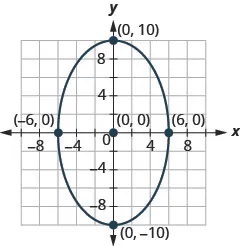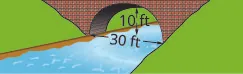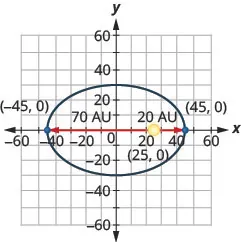Intermediate Algebra

# Practice Test

Intermediate AlgebraPractice Test

### Practice Test

In the following exercises, find the distance between the points and the midpoint of the line segment with the given endpoints. Round to the nearest tenth as needed.

327.

$(−4,−3)(−4,−3)$ and $(−10,−11)(−10,−11)$

328.

$(6,8)(6,8)$ and $(−5,−3)(−5,−3)$

In the following exercises, write the standard form of the equation of the circle with the given information.

329.

radius is 11 and center is $(0,0)(0,0)$

330.

radius is 12 and center is $(10,−2)(10,−2)$

331.

center is $(−2,3)(−2,3)$ and a point on the circle is $(2,−3)(2,−3)$

332.

Find the equation of the ellipse shown in the graph.In the following exercises, identify the type of graph of each equation as a circle, parabola, ellipse, or hyperbola, and graph the equation.

333.

$4 x 2 + 49 y 2 = 196 4 x 2 + 49 y 2 = 196$

334.

$y = 3 ( x − 2 ) 2 − 2 y = 3 ( x − 2 ) 2 − 2$

335.

$3 x 2 + 3 y 2 = 27 3 x 2 + 3 y 2 = 27$

336.

$y 2 100 − x 2 36 = 1 y 2 100 − x 2 36 = 1$

337.

$x 2 16 + y 2 81 = 1 x 2 16 + y 2 81 = 1$

338.

$x = 2 y 2 + 10 y + 7 x = 2 y 2 + 10 y + 7$

339.

$64 x 2 − 9 y 2 = 576 64 x 2 − 9 y 2 = 576$

In the following exercises, identify the type of graph of each equation as a circle, parabola, ellipse, or hyperbola, write the equation in standard form, and graph the equation.

340.

$25 x 2 + 64 y 2 + 200 x − 256 y − 944 = 0 25 x 2 + 64 y 2 + 200 x − 256 y − 944 = 0$

341.

$x 2 + y 2 + 10 x + 6 y + 30 = 0 x 2 + y 2 + 10 x + 6 y + 30 = 0$

342.

$x = − y 2 + 2 y − 4 x = − y 2 + 2 y − 4$

343.

$9 x 2 − 25 y 2 − 36 x − 50 y − 214 = 0 9 x 2 − 25 y 2 − 36 x − 50 y − 214 = 0$

344.

$y = x 2 + 6 x + 8 y = x 2 + 6 x + 8$

345.

Solve the nonlinear system of equations by graphing:
${3y2−x=0y=−2x−1.{3y2−x=0y=−2x−1.$

346.

Solve the nonlinear system of equations using substitution:
${x2+y2=8y=−x−4.{x2+y2=8y=−x−4.$

347.

Solve the nonlinear system of equations using elimination:
${x2+9y2=92x2−9y2=18.{x2+9y2=92x2−9y2=18.$

348.

Create the equation of the parabolic arch formed in the foundation of the bridge shown. Give the answer in $y=ax2+bx+cy=ax2+bx+c$ form.349.

A comet moves in an elliptical orbit around a sun. The closest the comet gets to the sun is approximately 20 AU and the furthest is approximately 70 AU. The sun is one of the foci of the elliptical orbit. Letting the ellipse center at the origin and labeling the axes in AU, the orbit will look like the figure below. Use the graph to write an equation for the elliptical orbit of the comet.350.

The sum of two numbers is 22 and the product is $−240.−240.$ Find the numbers.

351.

For her birthday, Olive’s grandparents bought her a new widescreen TV. Before opening it she wants to make sure it will fit her entertainment center. The TV is 55”. The size of a TV is measured on the diagonal of the screen and a widescreen has a length that is larger than the width. The screen also has an area of 1452 square inches. Her entertainment center has an insert for the TV with a length of 50 inches and width of 40 inches. What are the length and width of the TV screen and will it fit Olive’s entertainment center?

Order a print copy

As an Amazon Associate we earn from qualifying purchases.